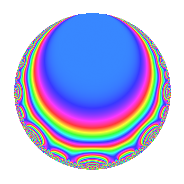# Properties

 Label 672.2.j.cLevel 672 Weight 2 Character orbit 672.j Analytic conductor 5.366 Analytic rank 0 Dimension 4 CM No Inner twists 2

# Related objects

## Newspace parameters

 Level: $$N$$ = $$672 = 2^{5} \cdot 3 \cdot 7$$ Weight: $$k$$ = $$2$$ Character orbit: $$[\chi]$$ = 672.j (of order $$2$$ and degree $$1$$)

## Newform invariants

 Self dual: No Analytic conductor: $$5.36594701583$$ Analytic rank: $$0$$ Dimension: $$4$$ Coefficient field: $$\Q(i, \sqrt{5})$$ Coefficient ring: $$\Z[a_1, \ldots, a_{7}]$$ Coefficient ring index: $$2$$ Sato-Tate group: $\mathrm{SU}(2)[C_{2}]$

## $q$-expansion

Coefficients of the $$q$$-expansion are expressed in terms of a basis $$1,\beta_1,\beta_2,\beta_3$$ for the coefficient ring described below. We also show the integral $$q$$-expansion of the trace form.

 $$f(q)$$ $$=$$ $$q + ( -\beta_{1} - \beta_{2} ) q^{3} + ( -\beta_{1} + \beta_{3} ) q^{5} -\beta_{2} q^{7} + ( -1 - \beta_{1} + 2 \beta_{2} + \beta_{3} ) q^{9} +O(q^{10})$$ $$q + ( -\beta_{1} - \beta_{2} ) q^{3} + ( -\beta_{1} + \beta_{3} ) q^{5} -\beta_{2} q^{7} + ( -1 - \beta_{1} + 2 \beta_{2} + \beta_{3} ) q^{9} + ( -2 \beta_{1} - 2 \beta_{2} - 2 \beta_{3} ) q^{11} + ( \beta_{1} + 2 \beta_{2} + \beta_{3} ) q^{13} + ( 2 - \beta_{1} + 2 \beta_{2} + \beta_{3} ) q^{15} -2 \beta_{2} q^{17} + ( -4 + \beta_{1} - \beta_{3} ) q^{19} + ( -1 + \beta_{3} ) q^{21} + ( -6 - 2 \beta_{1} + 2 \beta_{3} ) q^{23} + ( -1 - 2 \beta_{1} + 2 \beta_{3} ) q^{25} + ( 4 + 3 \beta_{2} - \beta_{3} ) q^{27} + ( 4 \beta_{1} + 4 \beta_{2} + 4 \beta_{3} ) q^{31} + ( -6 - 2 \beta_{1} + 4 \beta_{2} ) q^{33} + ( \beta_{1} + \beta_{3} ) q^{35} + ( 2 \beta_{1} + 2 \beta_{3} ) q^{37} + ( 4 + \beta_{1} - 2 \beta_{2} - \beta_{3} ) q^{39} + ( -2 \beta_{1} - 2 \beta_{2} - 2 \beta_{3} ) q^{41} + ( -6 - 2 \beta_{1} + 2 \beta_{3} ) q^{43} + ( 4 - 3 \beta_{1} - \beta_{3} ) q^{45} + ( 4 + 2 \beta_{1} - 2 \beta_{3} ) q^{47} - q^{49} + ( -2 + 2 \beta_{3} ) q^{51} + ( -4 + 2 \beta_{1} - 2 \beta_{3} ) q^{53} + ( -2 \beta_{1} + 8 \beta_{2} - 2 \beta_{3} ) q^{55} + ( -2 + 5 \beta_{1} + 2 \beta_{2} - \beta_{3} ) q^{57} + ( \beta_{1} + 2 \beta_{2} + \beta_{3} ) q^{59} + ( -3 \beta_{1} + 2 \beta_{2} - 3 \beta_{3} ) q^{61} + ( 2 + \beta_{1} + \beta_{2} + \beta_{3} ) q^{63} -4 \beta_{2} q^{65} + ( -2 - 4 \beta_{1} + 4 \beta_{3} ) q^{67} + ( 4 + 4 \beta_{1} + 10 \beta_{2} + 2 \beta_{3} ) q^{69} + ( -6 - 4 \beta_{1} + 4 \beta_{3} ) q^{71} + ( 6 + 2 \beta_{1} - 2 \beta_{3} ) q^{73} + ( 4 - \beta_{1} + 5 \beta_{2} + 2 \beta_{3} ) q^{75} + ( -2 - 2 \beta_{1} + 2 \beta_{3} ) q^{77} + ( -4 \beta_{1} - 8 \beta_{2} - 4 \beta_{3} ) q^{79} + ( 1 - 4 \beta_{1} - 4 \beta_{2} - 4 \beta_{3} ) q^{81} + ( \beta_{1} - 10 \beta_{2} + \beta_{3} ) q^{83} + ( 2 \beta_{1} + 2 \beta_{3} ) q^{85} -6 \beta_{2} q^{89} + ( 2 + \beta_{1} - \beta_{3} ) q^{91} + ( 12 + 4 \beta_{1} - 8 \beta_{2} ) q^{93} + ( -4 + 6 \beta_{1} - 6 \beta_{3} ) q^{95} + ( 10 + 2 \beta_{1} - 2 \beta_{3} ) q^{97} + ( 4 + 4 \beta_{1} + 10 \beta_{2} - 4 \beta_{3} ) q^{99} +O(q^{100})$$ $$\operatorname{Tr}(f)(q)$$ $$=$$ $$4q + 2q^{3} + 4q^{5} + O(q^{10})$$ $$4q + 2q^{3} + 4q^{5} + 12q^{15} - 20q^{19} - 2q^{21} - 16q^{23} + 4q^{25} + 14q^{27} - 20q^{33} + 12q^{39} - 16q^{43} + 20q^{45} + 8q^{47} - 4q^{49} - 4q^{51} - 24q^{53} - 20q^{57} + 8q^{63} + 8q^{67} + 12q^{69} - 8q^{71} + 16q^{73} + 22q^{75} + 4q^{81} + 4q^{91} + 40q^{93} - 40q^{95} + 32q^{97} + O(q^{100})$$

Basis of coefficient ring in terms of a root $$\nu$$ of $$x^{4} + 3 x^{2} + 1$$:

 $$\beta_{0}$$ $$=$$ $$1$$ $$\beta_{1}$$ $$=$$ $$\nu^{2} + \nu + 1$$ $$\beta_{2}$$ $$=$$ $$\nu^{3} + 2 \nu$$ $$\beta_{3}$$ $$=$$ $$-\nu^{2} + \nu - 1$$
 $$1$$ $$=$$ $$\beta_0$$ $$\nu$$ $$=$$ $$($$$$\beta_{3} + \beta_{1}$$$$)/2$$ $$\nu^{2}$$ $$=$$ $$($$$$-\beta_{3} + \beta_{1} - 2$$$$)/2$$ $$\nu^{3}$$ $$=$$ $$-\beta_{3} + \beta_{2} - \beta_{1}$$

## Character Values

We give the values of $$\chi$$ on generators for $$\left(\mathbb{Z}/672\mathbb{Z}\right)^\times$$.

 $$n$$ $$127$$ $$421$$ $$449$$ $$577$$ $$\chi(n)$$ $$-1$$ $$-1$$ $$-1$$ $$1$$

## Embeddings

For each embedding $$\iota_m$$ of the coefficient field, the values $$\iota_m(a_n)$$ are shown below.

For more information on an embedded modular form you can click on its label.

Label $$\iota_m(\nu)$$ $$a_{2}$$ $$a_{3}$$ $$a_{4}$$ $$a_{5}$$ $$a_{6}$$ $$a_{7}$$ $$a_{8}$$ $$a_{9}$$ $$a_{10}$$
239.1
 0.618034i − 0.618034i 1.61803i − 1.61803i
0 −0.618034 1.61803i 0 −1.23607 0 1.00000i 0 −2.23607 + 2.00000i 0
239.2 0 −0.618034 + 1.61803i 0 −1.23607 0 1.00000i 0 −2.23607 2.00000i 0
239.3 0 1.61803 0.618034i 0 3.23607 0 1.00000i 0 2.23607 2.00000i 0
239.4 0 1.61803 + 0.618034i 0 3.23607 0 1.00000i 0 2.23607 + 2.00000i 0
 $$n$$: e.g. 2-40 or 990-1000 Significant digits: Format: Complex embeddings Normalized embeddings Satake parameters Satake angles

## Inner twists

Char. orbit Parity Mult. Self Twist Proved
1.a Even 1 trivial yes
24.f Even 1 yes

## Hecke kernels

This newform can be constructed as the kernel of the linear operator $$T_{5}^{2} - 2 T_{5} - 4$$ acting on $$S_{2}^{\mathrm{new}}(672, [\chi])$$.